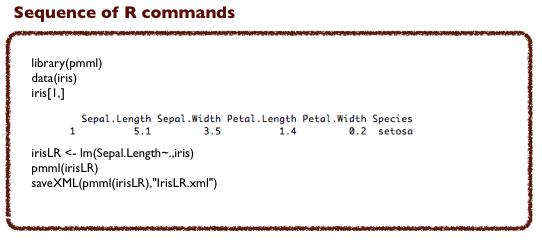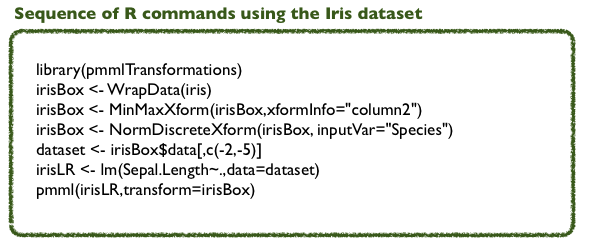### R and PMMLA PMML package for R that exports all kinds of predictive models is available directly from CRAN.
Traditionally, the pmml package offered support for the following data mining algorithms:
• ksvm (kernlab): Support Vector Machines
• nnet: Neural Networks
• rpart: C&RT Decision Trees
• lm & glm (stats): Linear and Binary Logistic Regression Models
• arules: Association Rules
• kmeans and hclust: Clustering Models
Recently, it has been expanded to support:
• multinom (nnet): Multinomial Logistic Regression Models
• glm (stats): Generalized Linear Models for classification and regression with a wide variety of link functions
• randomForest: Random Forest Models for classification and regression
• coxph (survival): Cox Regression Models to calculate survival and stratified cumulative hazards
• naiveBayes (e1071): Naive Bayes Classifiers
• glmnet: Linear ElasticNet Regression Models
The pmml package can also export data transformations built with the pmmlTransformations package (see below). It can also be used to merge two disctinct PMML files into one. For example, if transformations and model were saved into separate PMML files, it can combine both files into one, as described in Chapter 5 of the PMML book - PMML in Action.

### How does it work?

Simple, once you build your model using any of the supported model types, pass the model object as an input parameter to the pmml function as shown in the figure below:Example - sequence of R commands used to build a linear regression model using lm and the Iris dataset:### Documentation

For more on the pmml package, please take a look at the paper we published in The R Journal. For that, just follow the link below:
1) Paper: PMML: An Open Standard for Sharing Models
Also, make sure to check out the package's documentation from CRAN:
2) CRAN: pmml Package

## R PMML Transformations Package

This is a brand new R package. Called pmmlTranformations, this package transforms data and when used in conjunction with the pmml package, it allows for data transformations to be exported together with the predictive model in a single PMML file. Transformations currently supported are:
• Min-max normalization
• Z-score normalization
• Dummy-fication of categorical variables
• Value Mapping
• Discretization (binning)
• Variable renaming.

### How does it work?

The pmmlTransformations package works in tandem with the pmml package so that data pre-processing can be represented together with the model in the resulting PMML code.
In R, as shown in the figure below, this process includes three steps:
1. With the use of the pmmlTransformations package, transform the raw input data as appropriate
2. Use transformed and raw data as inputs to the modeling function/package (hclust, nnet, glm, ...)
3. Output the entire solution (data pre-processing + model) in PMML using the pmml packageExample - sequence of R commands used to build a linear regression model using lm with transformed data### Documentation

1) KDD Paper: The R pmmlTransformations Package
Also, make sure to check out the package's documentation from CRAN:
2) CRAN: pmmlTransformations Package